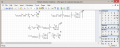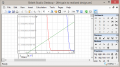#### tindel

Joined Sep 16, 2012
933
I am working on a RF(ish) receiver that uses a common source jFET input stage. The design will eventually be open source and I am planning on releasing detailed design information here in the completed projects section once I'm done. I am currently writing a report on how to design a CS input stage, and this has left me needing to be able to solve the following equation for Vgs... but I'm stuck - I don't even know where to look to try to solve this equation. You might notice that this equation is derived from the equation of the load line and the equation of the transconductance equal to each other.Note that sf is the 'squared factor' which is usually 2 - but does vary with transistor and I have accounted for this in my calculations. I could use the quadratic equation... Approximating the value by setting this value to 2, but this doesn't do me any good because there is no 'a' - not that I can readily identify anyway. I would also prefer to not assume a squared factor of 2.

Any help pointing me in the right direction would be helpful.

#### Jony130

Joined Feb 17, 2009
5,457
Last edited:

#### tindel

Joined Sep 16, 2012
933
But without assuming 'squared factor' it is impossible to solve this.
http://cecs.wright.edu/~dkender/bme3512/BiasingFETs.pdf (page 5)

Also I don't understand from where you get this part" 1/Rs*Vgs + Vgq/Rs" ?
Are you using the voltage divider at gate ??
Yes - I am biasing up the gate voltage to obtain less delta_I between transistor variance. That is where that term comes from.I guess I should clarify. I know the square factor - I have calculated it from data in the datasheet. I guess I'm asking how to solve this equation when the value is not 2. I've realized however that I'm not sure how to solve the equation if it was 2.

#### Papabravo

Joined Feb 24, 2006
20,400
Take the logarithm of both sides and solve the resulting equation.

#### Jony130

Joined Feb 17, 2009
5,457
Last edited: